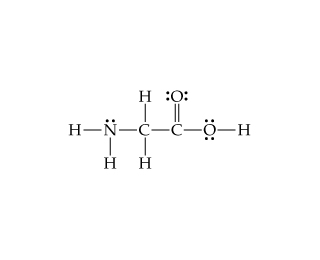# Problem: Consider the Lewis structure for glycine, the simplest amino acid: What are the approximate bond angles at the nitrogen?

###### FREE Expert Solution
99% (297 ratings)
###### FREE Expert Solution

We’re being asked to identify the bond angles around nitrogen in glycineSince the Lewis structure for glycine is already given, we simply need to do the following steps:

Step 1: Determine the number of electron groups around the indicated atom.

Step 2: Determine the electron geometry and bond angle using this:

Electron Regions          Electronic Geometry          Bond Angles

2                                      linear                                    180˚

3                                      trigonal planar                      120˚

4                                      tetrahedral                            109.5˚

5                                      trigonal bipyramidal              90˚, 120˚, and 180˚

6                                      octahedral                            90˚ and 180˚

99% (297 ratings)###### Problem Details

Consider the Lewis structure for glycine, the simplest amino acid:What are the approximate bond angles at the nitrogen?

What scientific concept do you need to know in order to solve this problem?

Our tutors have indicated that to solve this problem you will need to apply the Bond Angles concept. You can view video lessons to learn Bond Angles. Or if you need more Bond Angles practice, you can also practice Bond Angles practice problems.

What is the difficulty of this problem?

Our tutors rated the difficulty ofConsider the Lewis structure for glycine, the simplest amino...as medium difficulty.

How long does this problem take to solve?

Our expert Chemistry tutor, Sabrina took 3 minutes and 6 seconds to solve this problem. You can follow their steps in the video explanation above.

What professor is this problem relevant for?

Based on our data, we think this problem is relevant for Professor Dixon's class at UCF.